## The Matrix and Solving Systems with Matrices

Introduction to the matrix.

A matrix (plural matrices ) is sort of like a “table” of information where you are keeping track of things both right and left ( columns ), and up and down ( rows ). Usually, a matrix contains numbers or algebraic expressions . You may have heard matrices called arrays , especially in computer science.

As an example, if you had three sisters, and you wanted an easy way to store their age and number of pairs of shoes, you could store this information in a matrix. The actual matrix is inside and includes the brackets:

$\displaystyle \begin{array}{l}\,\,\,\,\,\,\,\,\,\,\,\,\,\,\,\,\,\,\,\,\,\,\,\,\,\,\,\,\,\,\,\,\,\,\,\,\,\,\,\,\,\,\,\,\,\,\,\,\,\,\,\,\,\,\,\,\,\,\begin{array}{*{20}{c}} {\text{Ashley}} & {\text{Emma}} & {\text{Chloe}} \end{array}\\\begin{array}{*{20}{c}} {\text{Age}} \\ {\text{Number of Pairs of Shoes}} \end{array}\text{ }\left[ {\begin{array}{*{20}{c}} {\text{23}} & {\,\,\,\,\,\,\,\,\,\text{18}} & {\,\,\,\,\,\,\,\,\,\text{15}} \\ \text{5} & {\,\,\,\,\,\,\,\,\text{23}} & {\,\,\,\,\,\,\,\,\,\text{12}} \end{array}} \right]\end{array}$

Matrices are called multi-dimensional since we have data being stored in different directions in a grid. The dimensions of this matrix are “ 2 x 3 ” or “ 2 by 3 ”, since we have 2 rows and 3 columns. (You always go down first , and then over to get the dimensions of the matrix). Each number or variable inside the matrix is called an entry or element , and can be identified by subscripts . For example, for the matrix above, “Ashley’s number of pairs of shoes ( 5 )” would be identified as ${{a}_{{2,1}}}$, since it’s on the 2 nd row and it’s the 1 st  entry. Again, matrices are great for storing numbers and variables – and also great for solving systems of equations, which we’ll see later. Note that we’ll address Solving Systems using   Reduced Row Echelon Form here .

Let’s look at a matrix that contains numbers and see how we can add and subtract matrices.

Let’s say you’re in avid reader, and in June, July, and August you read fiction and non-fiction books, and magazines, both in paper copies and online. You want to keep track of how many different types of books and magazines you read, and store that information in matrices.  Here is that information, and how it would look in matrix form:

Matrix Form:  $\left[ {\begin{array}{*{20}{c}} 2 & 4 \\ \begin{array}{l}3\\4\end{array} & \begin{array}{l}1\\5\end{array} \end{array}} \right]$

Matrix Form:  $\left[ {\begin{array}{*{20}{c}} 3 & 2 \\ \begin{array}{l}1\\5\end{array} & \begin{array}{l}1\\3\end{array} \end{array}} \right]$

Matrix Form:  $\left[ {\begin{array}{*{20}{c}} 1 & 3 \\ \begin{array}{l}2\\4\end{array} & \begin{array}{l}3\\6\end{array} \end{array}} \right]$

We can add matrices if the dimensions are the same; since the three matrices are all “ 3  by   2 ”, we can add them. For example, if we wanted to know the total number of each type of book/magazine we read, we could add each of the elements to get the sum:

$\displaystyle \require{cancel} \color{#800000}{{\left[ {\begin{array}{*{20}{c}} 2 & 4 \\ \begin{array}{l}3\\4\end{array} & \begin{array}{l}1\\5\end{array} \end{array}} \right]\,\,+\,\,\left[ {\begin{array}{*{20}{c}} 3 & 2 \\ \begin{array}{l}1\\5\end{array} & \begin{array}{l}1\\3\end{array} \end{array}} \right]\,\,\,+\,\,\left[ {\begin{array}{*{20}{c}} 1 & 3 \\ \begin{array}{l}2\\4\end{array} & \begin{array}{l}3\\6\end{array} \end{array}} \right]}}\,\,=\,\,\left[ {\begin{array}{*{20}{c}} {2+3+1} & {4+2+3} \\ \begin{array}{l}3+1+2\\4+5+4\end{array} & \begin{array}{l}1+1+3\\5+3+6\end{array} \end{array}} \right]\,\,=\,\,\left[ {\begin{array}{*{20}{c}} 6 & 9 \\ 6 & 5 \\ {13} & {14} \end{array}} \right]$

Thus we could see that we read 6 paper fiction, 9 online fiction, 6 paper non-fiction, 5 online non-fiction books, and 13 paper and 14 online magazines. We could also subtract matrices this same way. If we wanted to see how many books and magazines we would have read in August if we had doubled what we actually read, we could multiply the August matrix by the number 2 . This is called matrix scalar multiplication ; a scalar is just a single number that we multiply with every entry. Note that this is not the same as multiplying two matrices together (which we’ll get to next):

$\displaystyle \color{#800000}{{2\left[ {\begin{array}{*{20}{c}} 1 & 3 \\ \begin{array}{l}2\\4\end{array} & \begin{array}{l}3\\6\end{array} \end{array}} \right]}}\,=\,\left[ {\begin{array}{*{20}{c}} {1\times 2} & {3\times 2} \\ {2\times 2} & {3\times 2} \\ {4\times 2} & {6\times 2} \end{array}} \right]\,=\,\left[ {\begin{array}{*{20}{c}} 2 & 6 \\ 4 & 6 \\ 8 & {12} \end{array}} \right]$

## Multiplying Matrices

Multiplying matrices is a little trickier. First of all, you can only multiply matrices if the dimensions “match”; the second dimension (columns) of the first matrix has to match the first dimension (rows) of the second matrix , or you can’t multiply them. Think of it like the inner dimensions have to match , and the resulting dimensions of the new matrix are the outer dimensions .

Here’s an example of matrices with dimensions that would work. Again, always remember with matrices that you go down first and then over, to get their dimensions.Notice how the “middle” or “inner” dimensions of the first matrices have to be the same (in this case, “ 2 ”), and the new matrix has the “outside” or “outer” dimensions of the first two matrices (“ 3 by 5 ”).

Now, let’s do a real-life example to see how the multiplication works .   Let’s say we want to find the final grades for 3 girls, and we know what their averages are for tests, projects, homework, and quizzes. We also know that tests are 40% of the grade, projects 15% , homework 25% , and quizzes 20% . Here’s the data we have:

Let’s organize the following data into two matrices, and perform matrix multiplication to find the final grades for Alexandra, Megan, and Brittney; this is a weighted average ! To do this, you have to multiply in the following way:

Just remember when you put matrices together with matrix multiplication , the columns (what you see across) on the first matrix have to correspond to the rows down on the second matrix . You should end up with entries that correspond with the entries of each row in the first matrix. For example, with the problem above, the columns of the first matrix each had something to do with Tests, Projects, Homework, and Quizzes (grades). The row down on the second matrix each had something to do with the same four items (weights of grades). But then we ended up with information on the three girls (rows down on the first matrix), and the middle “descriptions” (test, projects, etc.) went away.

Alexandra has a 90 , Megan has a 77 , and Brittney has an 87 . See how cool this is? Matrices are really useful for a lot of applications in “real life”!

Now let’s do another example; let’s multiply the following matrices : (And don’t worry too much; most of the time you’ll be doing matrix multiplication in the calculator!)## Matrices in the Graphing Calculator

The TI graphing calculator is great for matrix operations! Here are some basic steps for storing, multiplying, adding, and subtracting matrices. (Note that you can also enter matrices using  ALPHA ZOOM  and the arrow keys in the newer graphing calculators, but I still like “naming” the matrices.)

We’ll learn other ways to use the calculator with matrices a little later.

## Determinants,  the Matrix Inverse, and the Identity Matrix

Soon we will be solving Systems of Equations using matrices, but we need to learn a few mechanics first!

Most square matrices (same dimension down and across) have what we call a determinant , designated by $\det \left[ \text{A} \right]$ or $\left| \text{A} \right|$, for example, which we’ll need to get the multiplicative inverse of that matrix. (The inverse of a matrix, designated by ${{\text{A}}^{{-1}}}$, for example, is what we multiply that square matrix by to get the identity matrix, a matrix with 1 ‘s along the diagonal starting with the upper left element, and 0 ‘s everywhere else.)

We’ll use the inverses of matrices to solve Systems of Equations ; the inverses will allow us to get variables by themselves on one side (like “regular” algebra). You’ll solve these mainly by using your calculator , but you’ll also have to learn how to get them “by hand”.

I know all this sounds confusing, but let’s do some examples step-by-step. Let’s first get the determinant of matrices (which we can get easily on a calculator!). The determinant is always just a scalar (number), and you’ll see it with two lines around the matrix:

Now let’s use the determinant to get the inverse of a matrix . We’ll only work with 2 by 2 matrices, since you’ll probably be able to use the calculator for larger matrices. Note again that only square matrices have inverses , but there are square matrices that don’t have one (when the determinant is 0 ):

Note that a matrix, multiplied by its inverse, if it’s defined, will always result in what we call an  Identity Matrix : $\displaystyle \left[ {\begin{array}{*{20}{c}} 3 & 1 \\ 4 & 8 \end{array}} \right]\,\times \,\left[ {\begin{array}{*{20}{c}} {\frac{2}{5}} & {-\frac{1}{{20}}} \\ {-\frac{1}{5}} & {\frac{3}{{20}}} \end{array}} \right]=\left[ {\begin{array}{*{20}{c}} 1 & 0 \\ 0 & 1 \end{array}} \right]$ . An identity matrix has 1 ’s along the diagonal starting with the upper left element, and 0 ’s everywhere else.

When you multiply a square matrix with an identity matrix, you just get that matrix back: $\displaystyle \left[ {\begin{array}{*{20}{c}} 3 & 1 \\ 4 & 8 \end{array}} \right]\,\times \,\left[ {\begin{array}{*{20}{c}} 1 & 0 \\ 0 & 1 \end{array}} \right]=\left[ {\begin{array}{*{20}{c}} 3 & 1 \\ 4 & 8 \end{array}} \right]$. Think of an  identity matrix  like “ 1 ” in regular multiplication (the multiplicative identity), and the  inverse matrix  like a  reciprocal  (the multiplicative inverse).

## Solving Systems with Matrices

Why are we doing all this crazy math? Because we can  solve systems  with the  inverse of a matrix , since the inverse is sort of like dividing to get the variables all by themselves on one side.

To solve systems with matrices, we use $\displaystyle X={{A}^{{-1}}}B$. Here is why, if you’re interested in the “theory” (the column on the right provides an example with “regular” multiplication). ( I  is the identity matrix.):

$\displaystyle \begin{array}{l}\,\,\,\,\,\,\,\,AX=B\,\,\,\,\,\,\,\,\,\,\,\,\,\,\,\,\,\,\,\,\,\,\,\,\,\,\,\,\,\,\,\,\,\,\,\,\,\,5x=10\\{{A}^{{-1}}}AX={{A}^{{-1}}}B\,\,\,\,\,\,\,\,\,\,\,\,\,\,\,\,\,\,\,\,\,\,\,\,\frac{1}{5}\cdot 5x=\frac{1}{5}\cdot 10\\\,\,\,\,\,\,\,\,\,\,IX={{A}^{{-1}}}B\,\,\,\,\,\,\,\,\,\,\,\,\,\,\,\,\,\,\,\,\,\,\,\,\,\,\,\,\,\,\,1x=2\\\,\,\,\,\,\,\,\,\,\,\,X={{A}^{{-1}}}B\,\,\,\,\,\,\,\,\,\,\,\,\,\,\,\,\,\,\,\,\,\,\,\,\,\,\,\,\,\,\,\,\,\,x=2\end{array}$

Here’s an example:

(It is important to note that if we are trying to solve a system of equations and the determinant turns out to be  0 , that system either has an  infinite number of solutions , or  no solution . See below. )

## Solving Systems with Reduced Row Echelon Form

There’s another way to solve systems by converting a systems’ matrix into reduced row echelon form , where we can put everything in one matrix (called an augmented matrix ). I show how to use this method by hand here in the Solving Systems using   Reduced Row Echelon Form section , but here I’ll just show you how to easy it is to solve using RREF in a graphing calculator :

A little easier, right?

## Solving Matrix Equations

Here are a couple more types of matrix problems you might see:

## Cramer’s Rule

Sometimes you’ll have to learn Cramer’s Rule , which is another way to solve systems with matrices. Cramer’s Rule was named after the Swiss mathematician Gabriel Cramer, who also did a lot of other neat stuff with math. Cramer’s rule is all about getting determinants of the square matrices that are used to solve systems. It’s really not too difficult; it can just be a lot of work, so again, I’ll take the liberty of using the calculator to do most of the work :). Let’s just show an example: Solve the following system using Cramer’s rule:     $\displaystyle \begin{array}{l}\,2x+3y-\,\,z\,=\,15\\4x-3y-\,\,z\,=\,19\\\,\,x\,-\,3y+\,3z\,=\,-4\end{array}$.

To solve for $x, y$, and $z$, we need to get the determinants of four matrices, the first one being the 3 by 3 matrix that holds the coefficients of $x,y$, and $z$. Let’s call this first determinant $D$;  $\displaystyle D=\det \left[ {\begin{array}{*{20}{c}} 2 & 3 & {-1} \\ 4 & {-3} & {-1} \\ 1 & {-3} & 3 \end{array}} \right]=-54$. Now get a matrix called ${{D}_{x}}$, which is obtained by “throwing away” the first ($x$) column, and replacing the numbers with the “answer” or constant column. Thus, $\displaystyle {{D}_{x}}=\det \left[ {\begin{array}{*{20}{c}} {\boldsymbol{{15}}} & 3 & {-1} \\ {\boldsymbol{{19}}} & {-3} & {-1} \\ {\boldsymbol{{-4}}} & {-3} & 3 \end{array}} \right]=-270$. You can probably guess what the next determinant we need is: ${{D}_{y}}$, which we get by “throwing away” the second column ($y$) of the original matrix and replacing the numbers with the constant column like we did earlier for the $x$. Thus, $\displaystyle {{D}_{y}}=\det \left[ {\begin{array}{*{20}{c}} 2 & {\boldsymbol{{15}}} & {-1} \\ 4 & {\boldsymbol{{19}}} & {-1} \\ 1 & {\boldsymbol{{-4}}} & 3 \end{array}} \right]=-54$. Similarly, $\displaystyle {{D}_{z}}=\det \left[ {\begin{array}{*{20}{c}} 2 & 3 & {\boldsymbol{{15}}} \\ 4 & {-3} & {\boldsymbol{{19}}} \\ 1 & {-3} & {\boldsymbol{{-4}}} \end{array}} \right]=108$. OK, now for the fun and easy part! To get the $x, y$, and $z$ answers to the system, you simply divide the determinants ${{D}_{x}}$, ${{D}_{y}}$, and ${{D}_{z}}$, by the determinant $D$, respectively. Thus, $\displaystyle \left[ {\begin{array}{*{20}{c}} x \\ \begin{array}{l}y\\z\end{array} \end{array}} \right]=\left[ {\begin{array}{*{20}{c}} {\tfrac{{{{D}_{x}}}}{D}} \\ {\tfrac{{{{D}_{y}}}}{D}} \\ {\tfrac{{{{D}_{z}}}}{D}} \end{array}} \right]=\left[ {\begin{array}{*{20}{c}} {\tfrac{{-270}}{{-54}}} \\ {\tfrac{{-54}}{{-54}}} \\ {\tfrac{{108}}{{-54}}} \end{array}} \right]=\left[ {\begin{array}{*{20}{c}} 5 \\ 1 \\ {-2} \end{array}} \right]$. Now we know that $x=5$, $y=1$, and $z=-2$. Note that, like the other systems, we can do this for any system where we have the same numbers of equations as unknowns .

## Number of Solutions when Solving Systems with Matrices

Most systems problems that you’ll deal with will just have one solution. (These equations are called independent or consistent). But, like we learned here in the Systems of Linear Equations and Word Problems  section , sometimes we have systems where we either have no solutions or an infinite number of solutions.

Without going too much into Geometry, let’s look at what it looks like when three systems (each system looks like a “plane” or a piece of paper) have an infinite number of solutions , no solutions , and one solution , respectively:Systems that have an infinite number of solution s (called dependent  or coincident ) will have two equations that are basically the same. One row of the coefficient matrix (and the corresponding constant matrix) is a multiple of another row. Thus, it’s like you’re trying to solve a system with only two equations, but three unknowns. A system that has an infinite number of solutions may look like this: \displaystyle \begin{align}2x+2y-z&=16\\4x+4y-2z&=32\\\,\,x-3y+3z&=-4\end{align} .

Systems with no solutions (called inconsistent ) will have one row of the coefficient matrix a multiple of another, but the rows in the constant matrix will not be in this same proportion. Thus, a system that has no solutions may look like this: \displaystyle \begin{align}2x+2y-z\,&=16\\4x+4y-2z&=10\\\,\,x\,-3y+3z&=-4\end{align} .## Applications of Matrices

Matrices can be used for many applications, including combining data, finding areas, and solving systems. Here are some examples of those applications; the most common is solving system of equations , as in the following problem:## Using the Determinant to get the Area of a Triangle

In your Geometry class, you may learn a neat trick where we can get the area of a triangle using the determinant of a matrix; let’s try an example. Let’s say we have the three coordinate points of that triangle, $\left( {{{a}_{1}},{{b}_{1}}} \right),\left( {{{a}_{2}},{{b}_{2}}} \right)$, and $\left( {{{a}_{3}},{{b}_{3}}} \right)$. The formula for the area of the triangle bounded by those points is:

$\displaystyle \text{Area of Triangle with points }\left( {{{a}_{1}},{{b}_{1}}} \right),\,\left( {{{a}_{2}},{{b}_{2}}} \right)\,\,\text{and}\,\left( {{{a}_{3}},{{b}_{3}}} \right)=\pm \frac{1}{2}\left| {\begin{array}{*{20}{c}} {{{a}_{1}}} & {{{b}_{1}}} & 1 \\ {{{a}_{2}}} & {{{b}_{2}}} & 1 \\ {{{a}_{3}}} & {{{b}_{3}}} & 1 \end{array}} \right|$ (Try both the plus and minus, but only take positive answer.)

## Matrix Multiplication Word Problem when Tables are Given

Here are some matrix multiplication applications:

The store wants to know how much their inventory is worth for all the shoes. How should we set up the matrix multiplication to determine this the best way? The trick for these types of problems is to line up what matches (flip flops, clogs, and Mary Janes), and that will be “ in the middle ” when we multiply (and these categories will disappear, which is OK). This way our dimensions will line up when combining the two tables.

Our matrix multiplication will look like this, even though our tables look a little different. We end up with numbers that relate to small, medium, and large shoes, so we can add up the amount in inventory (done on a calculator):We’ll have $1050 worth of small shoes,$2315 worth of medium shoes, and $1255 worth of large shoes for a total of$4620 . A nut distributor wants to know the nutritional content of various mixtures of almonds, cashews, and pecans. Her supplier has provided the following nutrition information:

Her first mixture, Mixture 1, consists of 6 cups of almonds, 3 cups of cashews, and 1 cup of pecans. Her second mixture, Mixture 2, consists of 3 cups of almonds, 6 cups of cashews, and 1 cup of pecans. Her third mixture, Mixture 3, consists of 3 cups of almonds, 1 cup of cashews, and 6 cups of pecans.

Determine the amount of protein, carbs, and fats in a 1 cup serving of each of the mixtures. Sometimes we can just put the information we have into matrices to sort of see what we are going to do from there. It makes sense to put the first group of data into a matrix with Almonds, Cashews, and Pecans as columns, and then put the second group of data into a matrix with information about Almonds, Cashews, and Pecans as rows. This way the columns of the first matrix lines up with the rows of the second matrix, and we can perform matrix multiplication. Then, we get rid of the number of cups of Almonds, Cashews, and Pecans , which we don’t need. Here is the second table we get from the problem:

Now we can multiply the matrices (using a graphing calculator) to end up with the amount of Protein, Carbs, and Fat (rows) in each of the mixtures (columns):

$\displaystyle \require{cancel} \begin{array}{l}\cancel{{\text{Almonds, Cashews and Pecans}}}\,\,\,\,\,\,\,\,\,\text{Mixture 1, Mixture 2, Mixture 3 }\,\,\,\,\,\,\,\,\,\,\,\,\,\,\,\text{Mixture 1, Mixture 2, Mixture 3}\\\,\,\begin{array}{*{20}{c}} {\text{Protein}} \\ {\text{Carbs}} \\ {\text{Fat}} \end{array}\,\,\,\left[ {\begin{array}{*{20}{c}} {26.2} & {21} & {10.1} \\ {40.2} & {44.8} & {14.3} \\ {71.9} & {63.5} & {82.8} \end{array}} \right]\,\,\,\,\,\,\times \,\,\,\cancel{{\begin{array}{*{20}{c}} {\text{Almonds}} \\ {\text{Cashews}} \\ {\text{Pecans}} \end{array}}}\,\,\,\,\,\left[ {\begin{array}{*{20}{c}} 6 & 3 & 3 \\ 3 & 6 & 1 \\ 1 & 1 & 6 \end{array}} \right]\,\,\,\,\,\,=\,\,\,\,\,\,\begin{array}{*{20}{c}} {\text{Protein}} \\ {\text{Carbs}} \\ {\text{Fat}} \end{array}\,\,\,\,\left[ {\begin{array}{*{20}{c}} {230.3} & {214.7} & {160.2} \\ {389.9} & {403.7} & {251.2} \\ {704.7} & {679.5} & {776} \end{array}} \right]\end{array}$

But we have to be careful, since these amounts are for 10 cups (add down to see we’ll get 10 cups for each mixture in the matrix above). Also, notice how the cups unit “canceled out” when we did the matrix multiplication (grams/cup times cups = grams). To get the answers, we have to divide each answer by 10 to get grams per cup . The numbers in bold are our answers:

Here are a few more matrix application problems that can get a little tricky:

## A Florist Must Make 5 Identical Bridesmaid Bouquets Systems Problem

Here’s a problem from the Systems of Linear Equations and Word Problems section ; we can see how much easier it is to solve with a matrix.

## An Input Output Problem

Input-output problems  are seen in Economics , where we might have industries that produce for consumers, but also consume for themselves. An application of matrices is used in this input-output analysis, which was first proposed by Wassily Leontief; in fact, he won the Nobel Prize in economics in 1973 for this work.

We can express the amounts (proportions) the industries consume in matrices, such as in the following problem. Don’t worry if you don’t get this now; I’m sure there are whole courses dedicated to this topic!

## Matrix Encoding/Decoding (Matrix Encryption) Problem

Matrix Encryption is a fun and interesting application; it involves using matrices in cryptology, where a (“secret”) encoded message can be sent to someone else (along with a random matrix) and decoded on the other end. Here’s an example:

a) For “ Math is Fun ”, (using 0 ’s for spaces), we have $13,1,20,8,0,9,19,0,6,21,14,0$; create matrix $\displaystyle M=\left[ {\begin{array}{*{20}{c}} {13} & 8 & {19} & {21} \\ 1 & 0 & 0 & {14} \\ {20} & 9 & 6 & 0 \end{array}} \right]$ (go down columns first). To encode, multiply the given matrix E by matrix M :

$\displaystyle EM=\left[ {\begin{array}{*{20}{c}} 2 & 4 & 1 \\ 3 & 9 & 5 \\ 6 & 8 & 0 \end{array}} \right]\times \left[ {\begin{array}{*{20}{c}} {13} & 8 & {19} & {21} \\ 1 & 0 & 0 & {14} \\ {20} & 9 & 6 & 0 \end{array}} \right]=\left[ {\begin{array}{*{20}{c}} {50} & {25} & {44} & {98} \\ {148} & {69} & {87} & {189} \\ {86} & {48} & {114} & {238} \end{array}} \right]$

Send the message as $50,148,86,25,69,48,44,87,114,98,189,238$ (go down columns first).

b) To decode the message, put the numbers received in matrix N and then compute ${{E}^{{-1}}}N$:

$\displaystyle {{E}^{{-1}}}N=\left[ {\begin{array}{*{20}{c}} {-4} & {.8} & {1.1} \\ 3 & {-.6} & {-.7} \\ {-3} & {.8} & {.6} \end{array}} \right]\times \left[ {\begin{array}{*{20}{c}} {50} & {25} & {44} & {98} \\ {148} & {69} & {87} & {189} \\ {86} & {48} & {114} & {238} \end{array}} \right]=\left[ {\begin{array}{*{20}{c}} {13} & 8 & {19} & {21} \\ 1 & 0 & 0 & {14} \\ {20} & 9 & 6 & 0 \end{array}} \right]$

Convert the numbers back into the letters (going down columns first) to get “ Math is Fun ”. Wow!

Learn these rules, and practice, practice, practice!

On to Solving Systems using Reduced Row Echelon Form  – you are ready!

## SOLVING WORD PROBLEMS USING INVERSE MATRIX

Problem 1 :find the products AB and BA and hence solve the system of equations x + y + 2z =1,3x + 2y + z = 7,2x + y + 3z = 2.AB  =  BA

x + y + 2z = 1, 3x + 2y + z = 7, 2x + y + 3z = 2|A|  =  1(6 - 1) - 1(9 - 2) + 2(3 - 4)

=  1(5) - 1(7) + 2(-1)

=  5 - 7 - 2

=  5 - 9

=  -4x  =  (-1/4)(-8)  =  2

y  =  (-1/4)(-4)  =  1

y  =  (-1/4)(4)  =  -1

So, the values of x, y and z area 2, 1 and -1 respectively.

Problem 2 :

A man is appointed in a job with a monthly salary of certain amount and a fixed amount of annual increment. If his salary was  ₹1 9,800 per month at the end of the first month after 3 years of service and  ₹ 23,400 per month at the end of the first month after 9 years of service, find his starting salary and his annual increment. (Use matrix inversion method to solve the problem.)

Let "x" and "y" be the  monthly salary and a fixed amount of annual increment respectively.

x + 3y  =  19800 ---------(1)

x + 9y  =  23400    ---------(2)|A|  =  9 - 3  =  6x  =  (178200 - 70200)/6  =  18000

y  =  (-19800 + 23400)/6  =  600

So, the monthly salary is 18000 and annual increment is 600.

Problem 3 :

Four men and 4 women can finish a piece of work jointly in 3 days while 2 men and 5 women  can finish the same work jointly in 4 days. Find the time taken by one man alone and that of  one woman alone to finish the same work by using  matrix inversion method.

Let "x" be the number of days taken by men and "y" be the number of days taken by women.

One day work done by 1 men  =  1/x

One day work done by 1 women  =  1/y

(4/x) + (4/y)  =  (1/3)

(2/x) + (5/y)  =  (1/4)

1/x  =  a, 1/y  =  b

4a + 4b  =  1/3  ---(1)

2a + 5b  =  1/4   ---(2)|A|  =  20 - 8  =  12x  =  18 and y = 36

So, men can take 18 days to finish the work and women can take 36 days to finish the work.

Problem 4 :

The prices of three commodities A,B and C are  ₹  x, y and z per units respectively. A person  P purchases 4 units of B and sells two units of A and 5 units of C . Person Q purchases 2  units of C and sells 3 units of A and one unit of B . Person R purchases one unit of A and  sells 3 unit of B and one unit of C . In the process, P,Q and R earn  ₹  15,000,  ₹  1,000 and  ₹ 4,000 respectively. Find the prices per unit of A,B and C . (Use matrix inversion method to  solve the problem.)

2x + 5z - 4y  =  15000

3x + y - 2z  =  1000

3y + z - x  =  4000

2x - 4y + 5z  =  15000  --------(1)

3x + y - 2z  =  1000   --------(2)

-x + 3y + z  =  4000   --------(3)|A|  =  2(1 + 6) + 4(3.- 2) + 5(9 + 1)

=  2(7) + 4(1) + 5(10)

=  14 + 4 + 50

|A|  =  68x  =   (1/68) (136000)  =  2000

y  =   (1/68) (68000)  =  1000

z  =   (1/68) (204000)  =  3000Apart from the stuff given above, if you need any other stuff in math, please use our google custom search here.

Kindly mail your feedback to   [email protected]

• Sat Math Practice
• SAT Math Worksheets
• PEMDAS Rule
• BODMAS rule
• GEMDAS Order of Operations
• Math Calculators
• Transformations of Functions
• Order of rotational symmetry
• Lines of symmetry
• Compound Angles
• Quantitative Aptitude Tricks
• Trigonometric ratio table
• Word Problems
• Times Table Shortcuts
• 10th CBSE solution
• PSAT Math Preparation
• Laws of Exponents

## Recent Articles## Eigenvalues of a Matrix

Oct 30, 23 09:23 PM

## Laws of Exponents Worksheet

Oct 30, 23 09:09 PM## Solving Multi Step Word Problems Worksheet

Oct 30, 23 09:06 PM

## Lesson Solving word problems by reducing to systems of linear equations in three unknowns#### IMAGES

1. How to Solve Word Problems with Matrices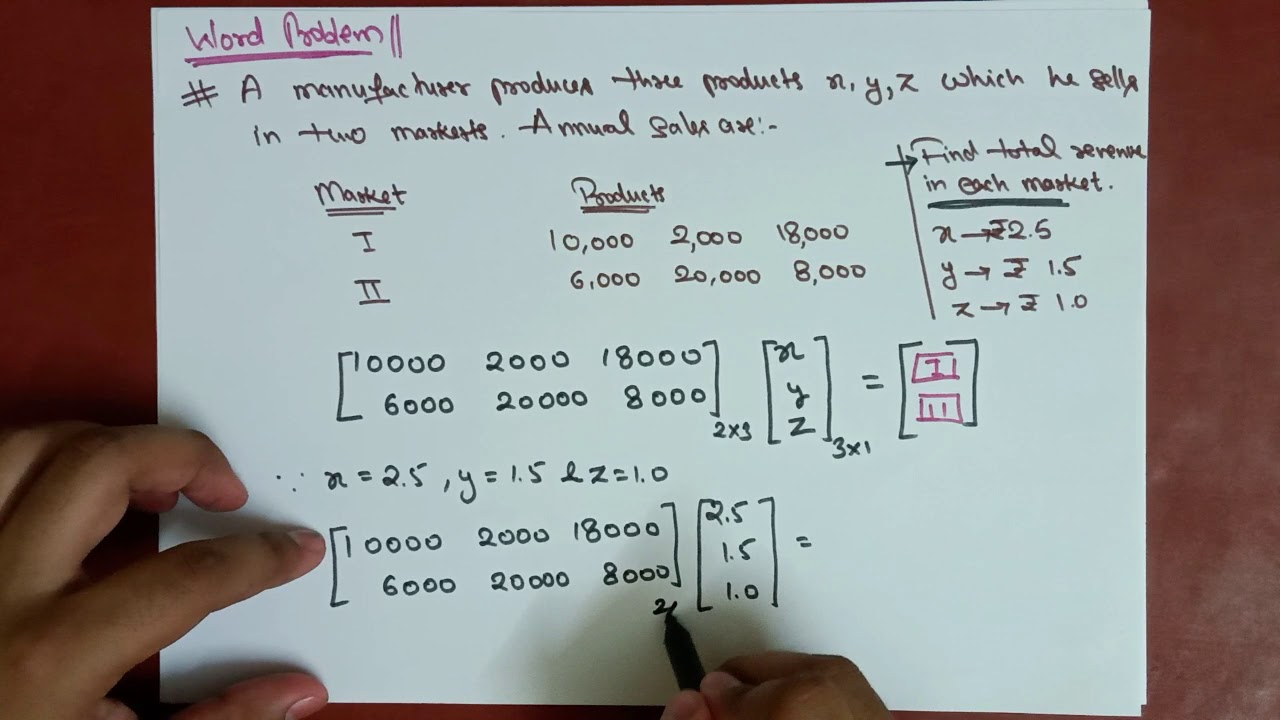2. Chapter 12-1 video 4: Using Matrices to Solve Word Problems (Matrix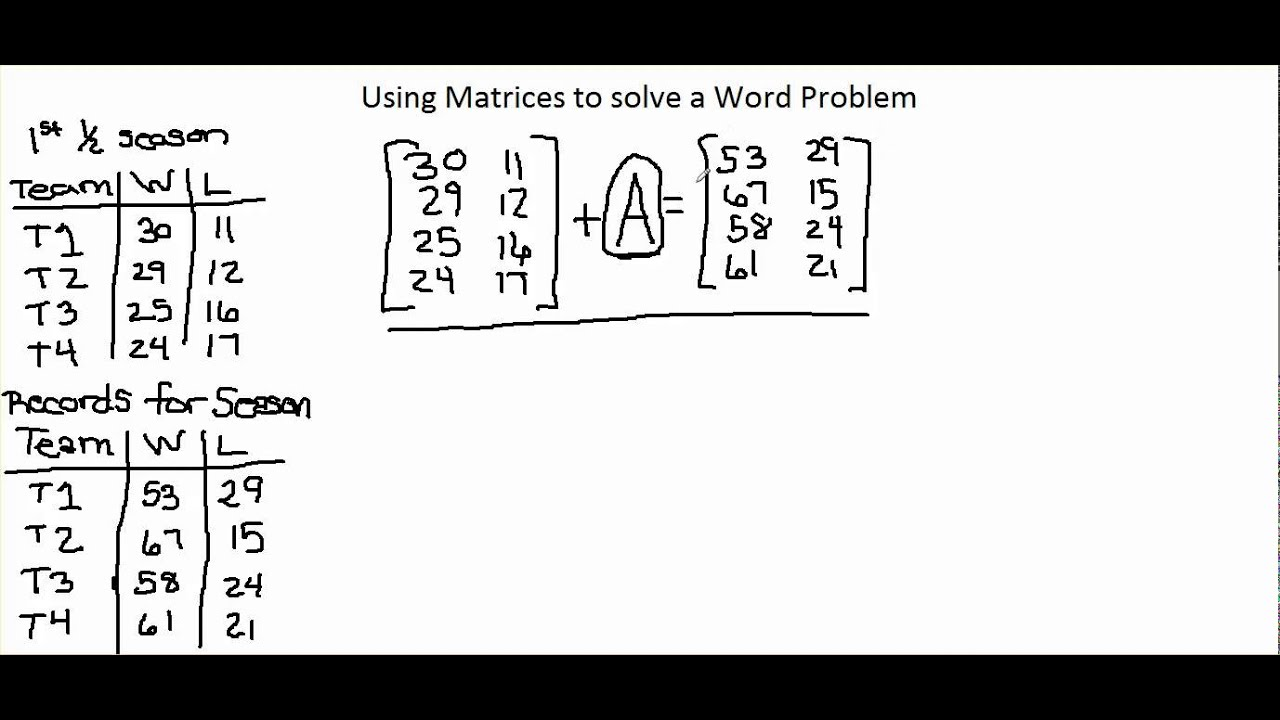3. Matrices Word Problems Worksheet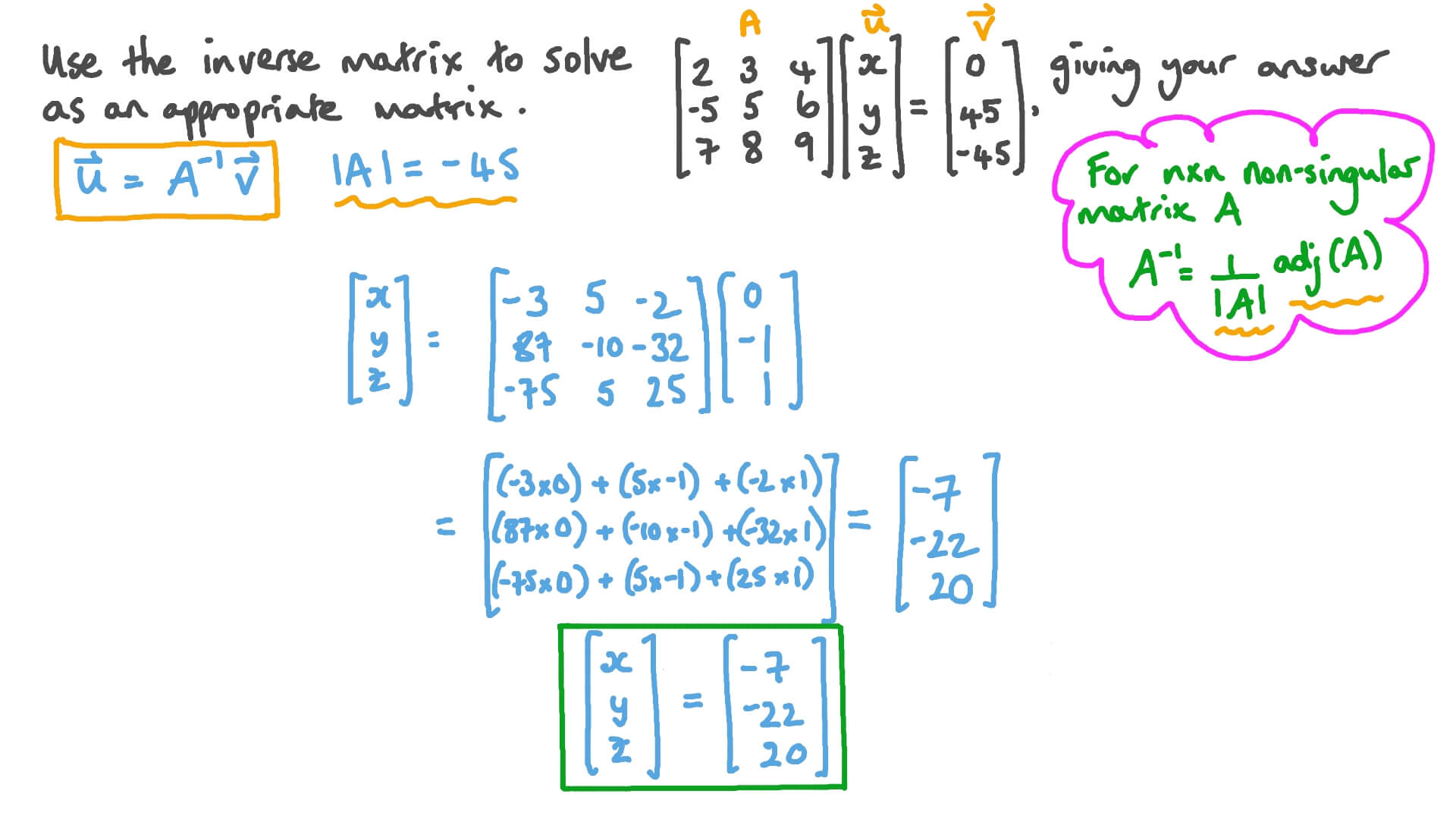4. Matrices Word Problems Worksheet5. Matrices Word Problems Worksheet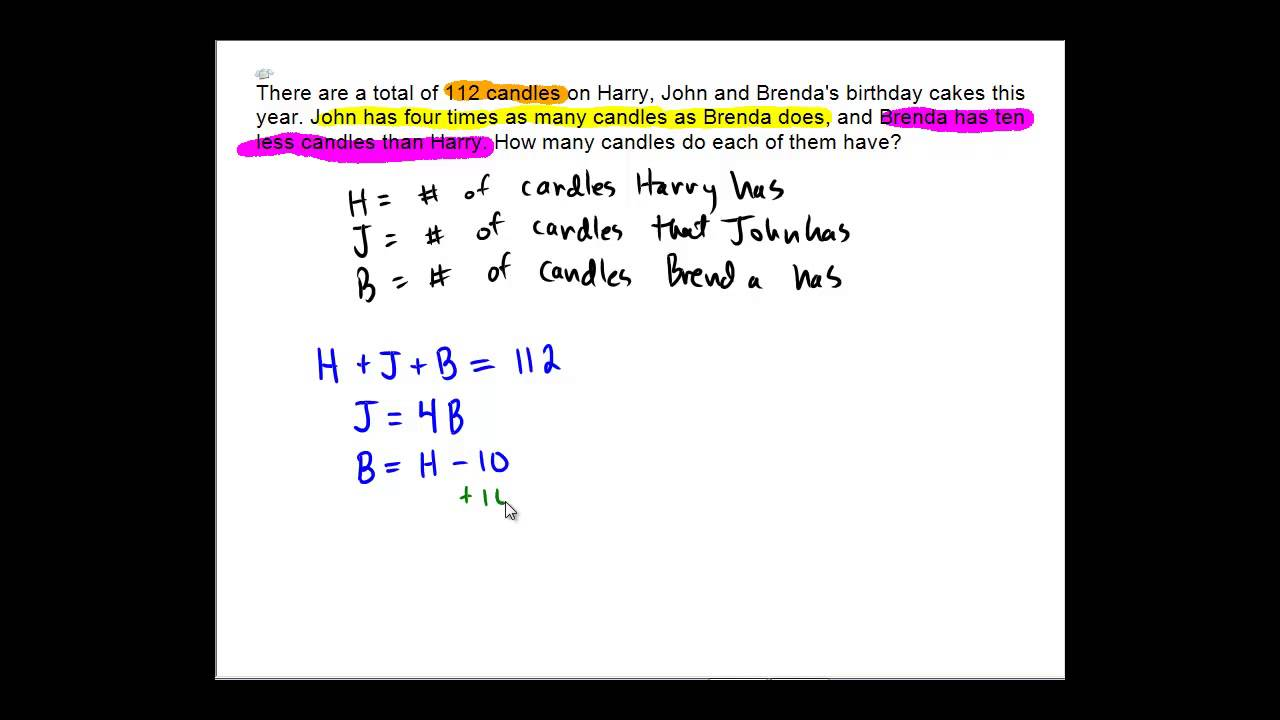6. Matrices Word Problems Worksheet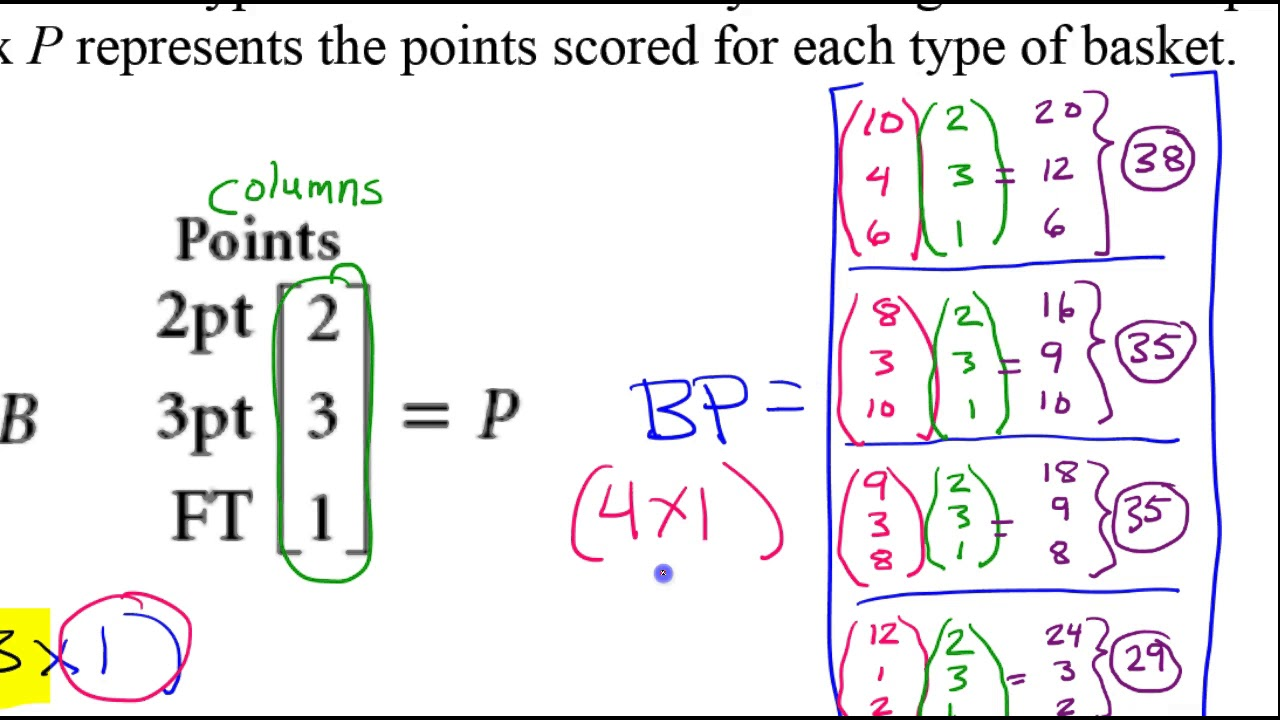#### VIDEO

1. Solving Problems Involving Matrices in Excel

2. Linear algebra matrices operations

3. Easiest way to solve matrices word problems /class 12

4. MATRICES MULTIPLICATION/MATRICES AND DETERMINANTS (PART 3(ii)/6) CLASS XII 12th CBSE

5. Day 08 (04 to 05) Using Matrices to Solve Word Problems

6. XII Maths : Unit 1 Ex 1.5 Word Problem Sum No 4

1. What Are Some Real Life Applications of Matrices?

Matrices, being the organization of data into columns and rows, can have many applications in representing demographic data, in computer and scientific applications, among others. They can be used as a representation of data or as a tool to...

2. Unlocking the Secrets of Math Homework: Expert Techniques to Solve Any Problem

Math homework can sometimes feel like an insurmountable challenge. From complex equations to confusing word problems, it’s easy to get overwhelmed. However, with the right techniques and strategies, you can conquer any math problem that com...

3. What Are the Six Steps of Problem Solving?

The six steps of problem solving involve problem definition, problem analysis, developing possible solutions, selecting a solution, implementing the solution and evaluating the outcome. Problem solving models are used to address issues that...

4. How to Solve a System of Equations Word Problem Using Matrices

http://www.mathproblemgenerator.com - How to Solve a System of Equations Word Problem Using Matrices. For more practice and to create math

5. Matrix word problem: prices (video)

The whole point of matrices is that we can use them to solve systems that have many equations. So rather than solving one by one, there are operations that

6. Day 08 (01) Using Matrices to Solve Word Problems (Mostly by Hand)

Share your videos with friends, family, and the world.

7. The Matrix and Solving Systems with Matrices

... matrix application problems that can get a little tricky: Matrix Multiplication Word Problem, Solution. Problem where no tables are given: Several years ago

8. Matrix Word Problems

Over 2000 math exercises. Matrix word problems. Solve the matrix word problems on Math-Exercises.com - Collection of math problems & math exercises.

9. Solving Linear Systems Using Matrices and Technology

For the following word problems, set up a system of linear equations to solve using matrices. A mix of 1 lb almonds and 1.5 lbs cashews

10. Solving Word Problems by Applying Operations on Matrices

The table shows the number of different types of rooms in three hotels owned by a company. If a single room costs 160 LE per night

11. A baseball souvenir shop is offering the following packages for

Matrix Word Problems. 1. A landscaping company placed two orders with a

12. Solving Word Problems Using Inverse Matrix

A person P purchases 4 units of B and sells two units of A and 5 units of C . Person Q purchases 2 units of C and sells 3 units of A and one unit of B . Person

13. Implementing a matrix that describes the word problem

In order to solve the question, I think it is safe to assume that every car available at each location is in fact rented each day. M2

14. Solving word problems by reducing to systems of linear equations in

(Again, I used the solver Finding the Determinant of a 3x3 Matrix to calculate the determinants). Thus the solution is x = Dx%2FD = 7200%2F40 = 180 , y = Dy%2FD